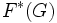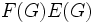# Generalized Fitting subgroup

Jump to: navigation, search
This article defines a subgroup-defining function, viz., a rule that takes a group and outputs a unique subgroup
View a complete list of subgroup-defining functions OR View a complete list of quotient-defining functions

This article is about a definition in group theory that is standard among the group theory community (or sub-community that dabbles in such things) but is not very basic or common for people outside.
VIEW: Definitions built on this | Facts about this: (facts closely related to Generalized Fitting subgroup, all facts related to Generalized Fitting subgroup) |Survey articles about this | Survey articles about definitions built on this
VIEW RELATED: Analogues of this | Variations of this | Opposites of this |
View a list of other standard non-basic definitions

This article is about a term related to the Classification of finite simple groups

## Definition

### Symbol-free definition

The generalized Fitting subgroup of a group is defined as the product of its Fitting subgroup with its layer (the layer here is the commuting product of all the components).

### Definition with symbols

Let$G$ be a group. The generalized Fitting subgroup of$G$, denoted as$F^*(G)$, is defined as the product$F(G)E(G)$, where$F(G)$ is the Fitting subgroup and$E(G)$ is the layer.